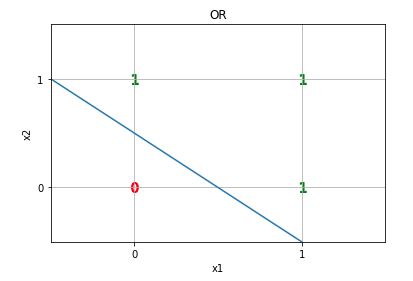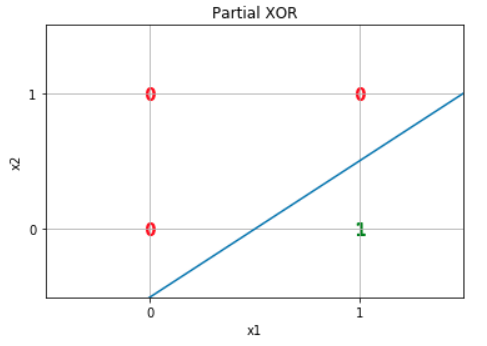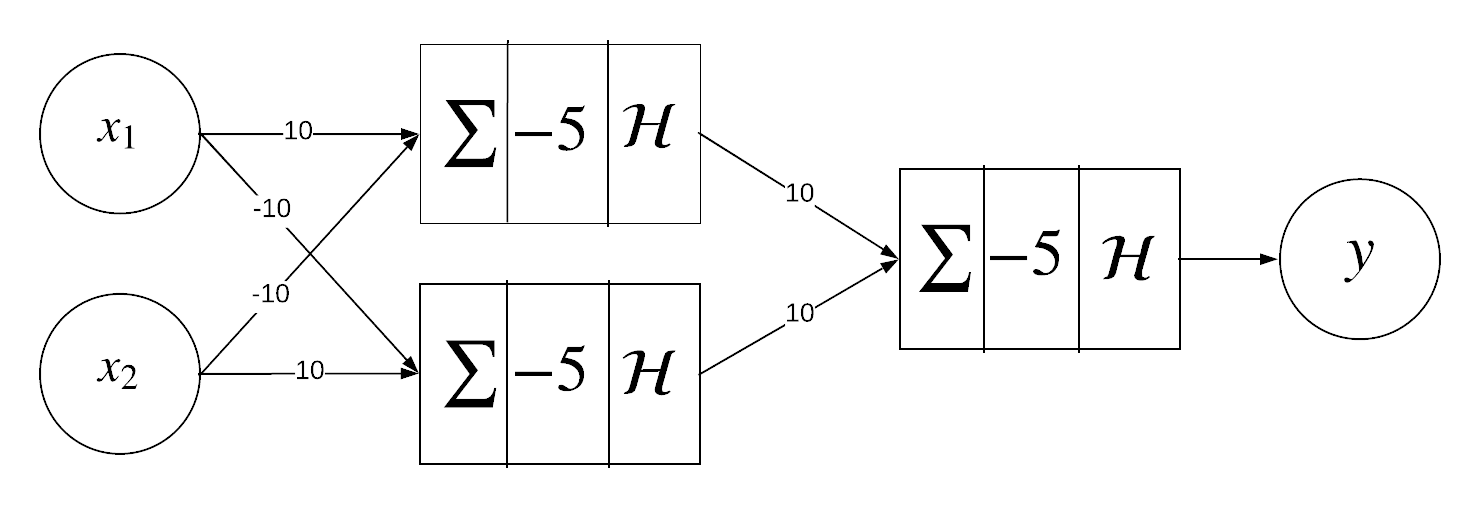Hello humans, by now you should know what the heck is a perceptron : a way to find the parameters w and b of a binary linear classifer. Recall that we will be using the following definition of a b.l.c :

$y = \mathcal{H}(\mathbf{x} \cdot \mathbf{w}-b)$

With a perceptron we can easily find appropriate values of b and w to compute many boolean functions. For example, we can do it easily with the “OR” function :

$x_1\vee x_2 \text{ also noted } x_1 + x_2$

The “OR” function has the following truth table :

x1 x2 x1 + x2
1 1 1
1 0 1
0 1 1
0 0 0

If we plot these values, we can see that we can separate them by a line :So, we have binary values and they are linearly separable, therefore we can implement this function using a perceptron by setting :

$\mathbf{w}=\begin{pmatrix}10\\10\end{pmatrix}\text{ and }b= 5 \\ x_1\vee x_2=\mathcal{H}(10\cdot x_1+10\cdot x_2-5)$

(Note that we could have used other values). Just to make sure it works, let’s manually compute the result :

x1 x2 10*x1 + 10*x2 - 5 H
1 1 15 1
1 0 5 1
0 1 5 1
0 0 -5 0

Ok, nice, but what about the XOR function ?

x1 x2 x1 xor x2
1 1 0
1 0 1
0 1 1
0 0 0

Try to separate these points by a single line…Yep, you can’t. Perceptrons can only classify linearly separable data.

But let’s try to be a little bit smart and go back to the definition of the “Xor” :

$x_1 \oplus x_2 = (x_1 \wedge \neg x_2) \vee (\neg x_1 \wedge x_2)$

The xor function is made of 3 basic operations : one OR, and two ANDs. The two ANDs are really similar, let’s call them “partial xor”.If we focus on one of the partial xor, we can write :

$\text{Partial xor = }x_1 \wedge \neg x_2$

This function has the following truth table :

x1 x2 x1 Pxor x2
1 1 0
1 0 1
0 1 0
0 0 0

If we plot it, we can see that we can separate the true value from the false by a line.We can use a perceptron, if we set (for example) :

$\mathbf{w}=\begin{pmatrix}10\\-10\end{pmatrix}\text{ and }b= 5$

If we want to use the same weight vector to implement the second partial xor, we just need to switch the rows of x1 and x2 in x :

$\mathbf{x}=\begin{pmatrix}x_2\\x_1\end{pmatrix}$

Now that we have a way to represent these 3 operations with perceptrons. We can link them together and build a 2-layer perceptron :By building a multilayer percetron, we can classify non-linearly separable data.

what’s next

The last 3 articles were just an introduction to the subject of neural networks. They will help us understand what neurons are, how they work, why we use multilayers neural networks.

Let’s gooo